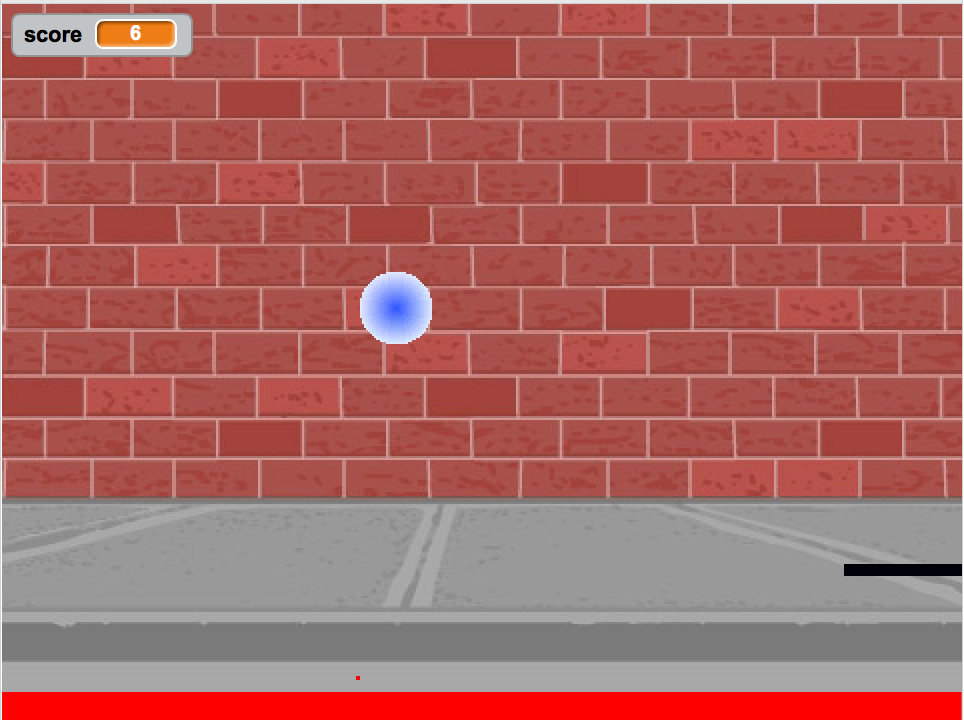3.1. What is a Variable?¶

The following video is also on YouTube at https://youtu.be/pHgYlVjagmA. It explains what a variable is and gives a couple of real word examples of variables.

A variable is a name associated with a memory location in the computer. Computer memory can store a value and that value can change or vary. When you play a game, it will often have a score. Scores often start at 0 and increase. A score is a variable.Figure 1: A pong game in Scratch with a score shown in the upper left.

3.1.1. Variable Types on the Exam¶

There are two types of variables in Java: primitive variables that hold primitive types and object variables that hold a reference to an object of a class. A reference is a way to find the object (like a UPS tracking number helps you find your package). The primitive types on the Advanced Placement Computer Science A exam are:

• int - which store integers (whole numbers like 3, -76, 20393)
• double - which store floating point numbers (decimal numbers like 6.3 -0.9, and 60293.93032)
• boolean - which store Boolean values (either true or false).

`String` is one of the object types on the exam and is the name of a class in Java. A string object has a sequence of characters enclosed in a pair of double quotes - like “Hello”. You will learn more about `String` objects in another chapter.

Note

Some languages use 0 to represent false and 1 to represent true, but Java uses the keywords `true` and `false`.

3-1-1: What type should you use to represent the average grade for a course?
• int
• While you could use an int, this would throw away any digits after the decimal point, so it isn't the best choice. You might want to round up a grade based on the average (89.5 or above is an A).
• double
• An average is calculated by summing all the values and dividing by the number of values. To keep the most amount of information this should be done with decimal numbers so use a double.
• boolean
• Is an average true or false?
• String
• While you can use a string to represent a number, using a number type (int or double) is better for doing calculations.
3-1-2: What type should you use to represent the number of people in a household?
• int
• The number of people is a whole number so using an integer make sense.
• double
• Can you have 2.5 people in a household?
• boolean
• Is the number of people something that is either true or false?
• String
• While you can use a string, a number is better for doing calculations with (like finding the average number of people in a household).
3-1-3: What type should you use to hold the first name of a person?
• int
• People don't usually have whole numbers like 7 as their first name.
• double
• People don't usually have decimal numbers like 3.5 as their first name.
• boolean
• This could only be used if the name was true or false. People don't usually have those as first names.
• String
• Strings hold sequences of characters like you have in a person's name.
3-1-4: What type should you use to record if it is raining or not?
• int
• While you could use an int and use 0 for false and 1 for true this would waste 31 of the 32 bits an int uses. Java has a special type for things that are either true or false.
• double
• Java has a special type for variables that are either true or false.
• boolean
• Java uses boolean for values that are only true or false.
• String
• While you can use a string to represent "True" or "False", using a boolean variable would be better for making decisions.
3-1-5: What type should you use to represent the amount of money you have?
• int
• The integer type (int) can't be used to represent decimal numbers so you couldn't use it if you had any cents.
• double
• The double type can be used to represent an amount of money.
• boolean
• Java uses boolean for values that are only true or false.
• String
• While you can use a string to represent the amount of money you have it is easier to do calculations on the numeric types (int or double).
Next Section - 3.2. Declaring Variables in Java